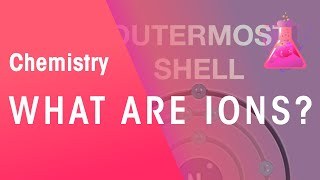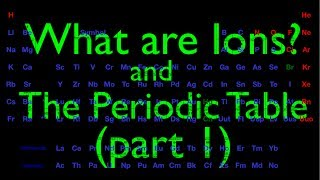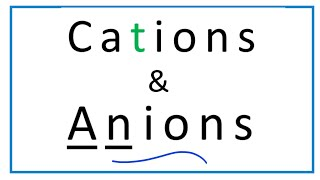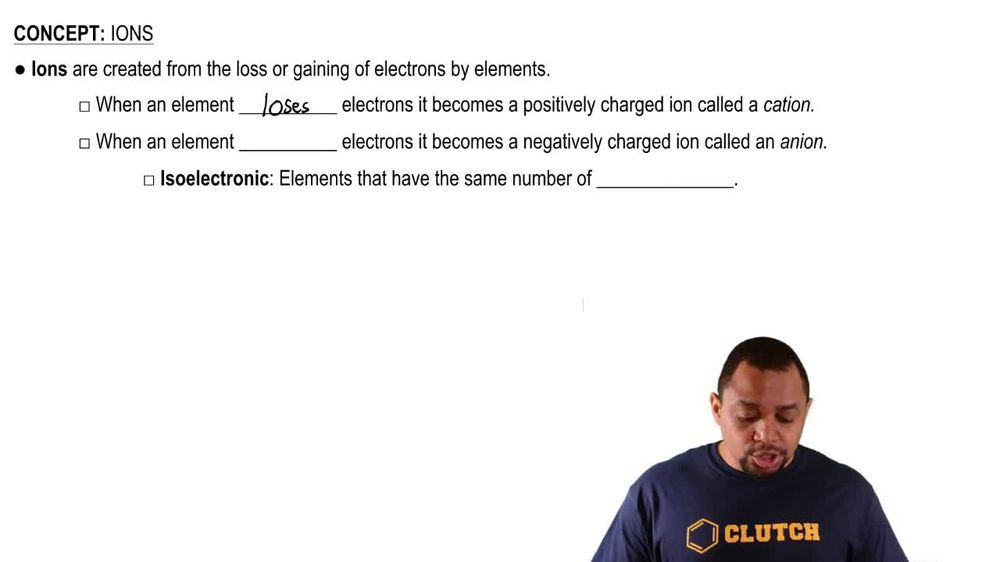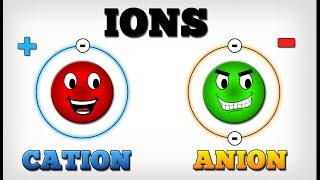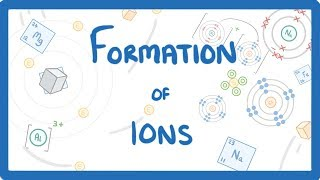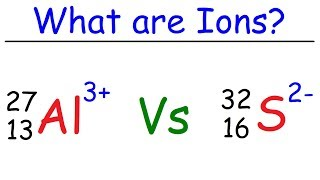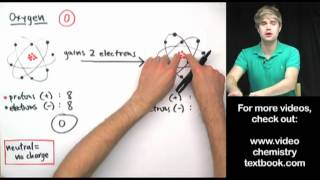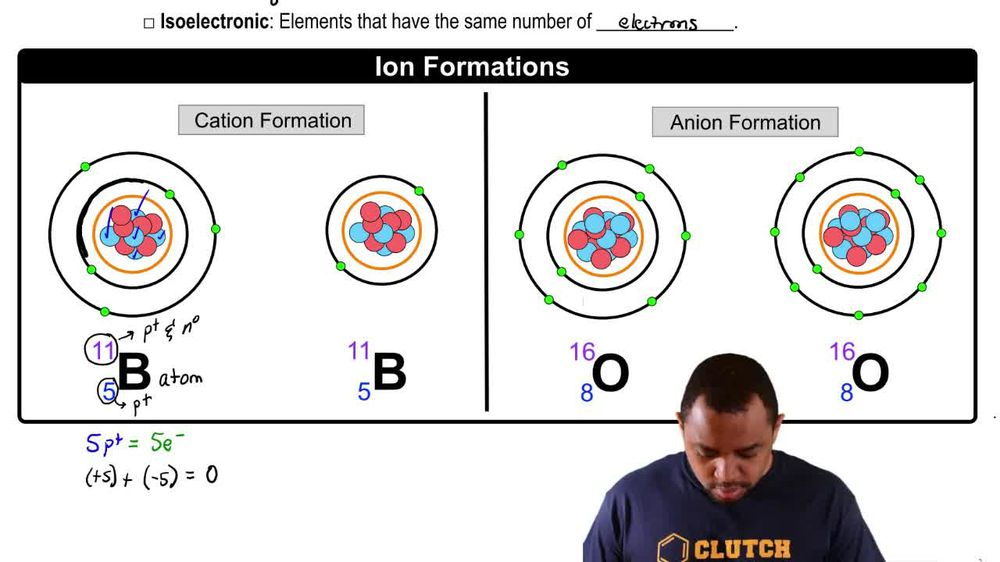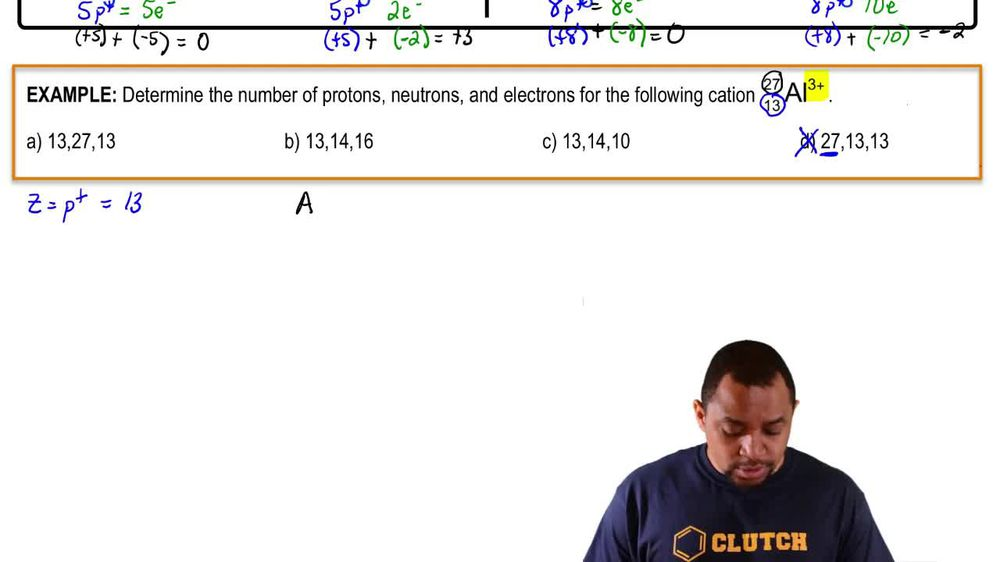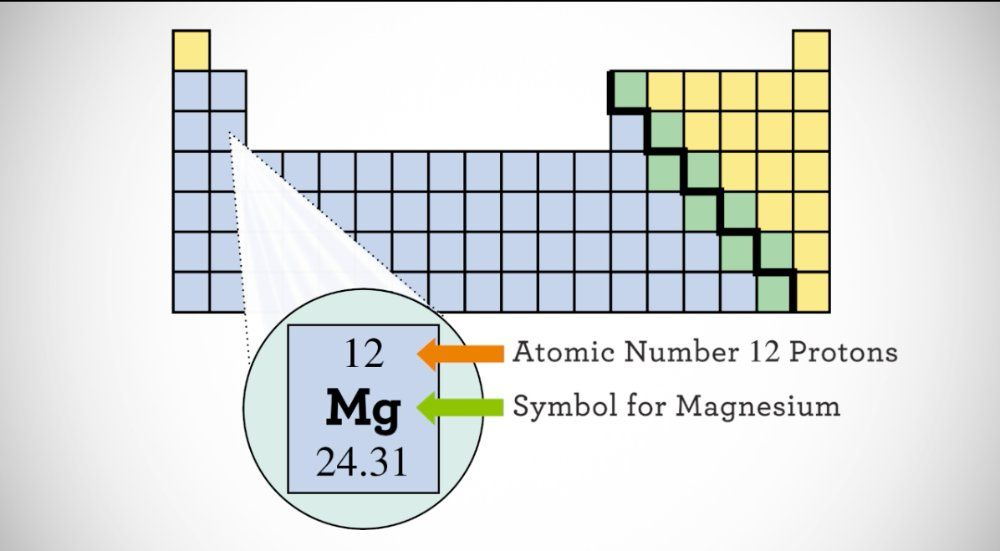Start typing, then use the up and down arrows to select an option from the list.
1. 2. Atoms & Elements2. Ions# Ions Example 1

by Jules Bruno
136 views
1
1
So here in this example question, it states determine the number of protons, neutrons and electrons for the following cat ion. Now it's a cat ion because it possesses a positive charge, that positive charge being three plus now, when looking at the other numbers, we know that this 13 here represents our atomic number, which uses the variables. E remember the atomic number gives us the number of protons, and because it's 13, that means we have 13 protons. That means that option. He cannot be an answer, because here we do not have 27 protons. Next, we have the number above the 13 and 27. Remember, that is your mass number, which uses the variable A. Your mass number gives you the number of protons plus neutrons within the element or ion. From this information, we know that the number off neutrons would equal a minus Z, so it's the mass number minus the atomic number, So that would be 27 minus 13, which would give us 14 neutrons. So so far, options B and C can be the only correct choices because in a we do not have 27 new trials. Now the charge is three plus three plus means that you have lost electrons. It means you have lost three electrons. When aluminum is neutral has no charge. It has an equal number of protons and electrons. Okay, but now we've lost three electrons. So what does that mean? That means we have 10 electrons remaining. So the answer would have to be see here. And if you want to double check that, you could just say we have 13 protons and 10 electrons, so we know that would be plus 13 plus minus 10, which would give us plus three as an answer. That proves that our charge should be plus three here. So, out of all the choices present Onley option C gives the correct number for each of the subatomic particles.